##### The Japanese Maple specialist
Direct order Contact Help / Services Newsletter# Shelf to displaySearch

Japanese MaplesYoung trees Acer seeds and more Bonsai soil and fertiliserVarieties introduced into EuropeHostasWind bells and chimesSaxifragesSpecial offer

Packing and shipping charges

# Shigi no hoshi

› Japanese maples › Acer matsumurae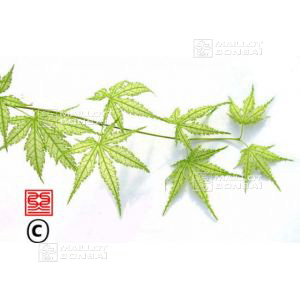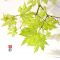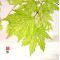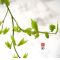ref. : 921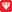Choose the right size
Pot size

This item is temporarily unavailable

###### Description

Leaves: tender green in spring, and then yellow or light green streaked with dark green, in autumn the leaves turn yellow.

#green 3.5 #matsumurae 3 #leaves 2.6 #yellow 2.6 #hoshi 2.5 #shigi 2.5 #acer 2.4 #streaked 1.8 #autumn 1.6 #spring 1.6

###### Technical description
 Species Acer matsumurae same species items Variety Shigi no hoshi Leaf colour Veined same colour items Leaf shape Palmated same shaped items Adult height same height items Port Natural same port items Expositions Sun same exposition items

Formule
(( ROUND((CHAR_LENGTH(b.article_nom)-CHAR_LENGTH(REPLACE(b.article_nom, 'green', '')))/LENGTH('green')) + ROUND((CHAR_LENGTH(b.article_description)-CHAR_LENGTH(REPLACE(b.article_description, 'green', '')))/LENGTH('green')) ) * 3.5) + (( ROUND((CHAR_LENGTH(b.article_nom)-CHAR_LENGTH(REPLACE(b.article_nom, 'leaves', '')))/LENGTH('leaves')) + ROUND((CHAR_LENGTH(b.article_description)-CHAR_LENGTH(REPLACE(b.article_description, 'leaves', '')))/LENGTH('leaves')) ) * 2.6) + (( ROUND((CHAR_LENGTH(b.article_nom)-CHAR_LENGTH(REPLACE(b.article_nom, 'yellow', '')))/LENGTH('yellow')) + ROUND((CHAR_LENGTH(b.article_description)-CHAR_LENGTH(REPLACE(b.article_description, 'yellow', '')))/LENGTH('yellow')) ) * 2.6) + (( ROUND((CHAR_LENGTH(b.article_nom)-CHAR_LENGTH(REPLACE(b.article_nom, 'hoshi', '')))/LENGTH('hoshi')) + ROUND((CHAR_LENGTH(b.article_description)-CHAR_LENGTH(REPLACE(b.article_description, 'hoshi', '')))/LENGTH('hoshi')) ) * 2.5) + (( ROUND((CHAR_LENGTH(b.article_nom)-CHAR_LENGTH(REPLACE(b.article_nom, 'shigi', '')))/LENGTH('shigi')) + ROUND((CHAR_LENGTH(b.article_description)-CHAR_LENGTH(REPLACE(b.article_description, 'shigi', '')))/LENGTH('shigi')) ) * 2.5) + (( ROUND((CHAR_LENGTH(b.article_nom)-CHAR_LENGTH(REPLACE(b.article_nom, 'streaked', '')))/LENGTH('streaked')) + ROUND((CHAR_LENGTH(b.article_description)-CHAR_LENGTH(REPLACE(b.article_description, 'streaked', '')))/LENGTH('streaked')) ) * 1.8) + (( ROUND((CHAR_LENGTH(b.article_nom)-CHAR_LENGTH(REPLACE(b.article_nom, 'autumn', '')))/LENGTH('autumn')) + ROUND((CHAR_LENGTH(b.article_description)-CHAR_LENGTH(REPLACE(b.article_description, 'autumn', '')))/LENGTH('autumn')) ) * 1.6) + (( ROUND((CHAR_LENGTH(b.article_nom)-CHAR_LENGTH(REPLACE(b.article_nom, 'spring', '')))/LENGTH('spring')) + ROUND((CHAR_LENGTH(b.article_description)-CHAR_LENGTH(REPLACE(b.article_description, 'spring', '')))/LENGTH('spring')) ) * 1.6) + (( ROUND((CHAR_LENGTH(b.article_nom)-CHAR_LENGTH(REPLACE(b.article_nom, 'tender', '')))/LENGTH('tender')) + ROUND((CHAR_LENGTH(b.article_description)-CHAR_LENGTH(REPLACE(b.article_description, 'tender', '')))/LENGTH('tender')) ) * 1.6) + (( ROUND((CHAR_LENGTH(b.article_nom)-CHAR_LENGTH(REPLACE(b.article_nom, 'light', '')))/LENGTH('light')) + ROUND((CHAR_LENGTH(b.article_description)-CHAR_LENGTH(REPLACE(b.article_description, 'light', '')))/LENGTH('light')) ) * 1.5)

## Secure payment## Delivery

Our logistic partners :04 74 55 23 48
Pépinière MAILLOT-BONSAÏ
Le Bois Frazy
01990 RELEVANT - FRANCE
on appointmentThe Japanese Maple specialist
If you like Maillot Bonsaï, share on  facebook

Pensez à venir nous rendre visite sur rendez vous. 04 74 55 23 48 merci.

L'équipe Maillot bonsaï vous souhaite ses meilleurs vœux de bonheur, de santé et de réussite pour cette nouvelle année. C'est l'occasion pour nous de vous dire un IMMENSE MERCI d'avoir été à nos côtés cette année.

2023, c'est 365 jours où nous serons présents pour vous aider, vous guider et répondre à vos questions. Plus que jamais, nous continuerons à vous accompagner et à vous proposer des produits de qualité.   Cette nouvelle année sera riche en nouveautés, alors restez connecté pour ne rien rater !

Guy Maillot

OK